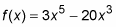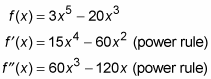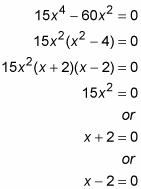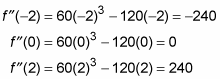##### Calculus II Workbook For DummiesThe Second Derivative Test is based on two prize-winning ideas: First, that at the crest of a hill, a road has a hump shape — in other words, it’s curving down or concave down. And second, at the bottom of a valley, a road is cup-shaped, so it’s curving up or concave up.

The concavity of a function at a point is given by its second derivative: A positive second derivative means the function is concave up, a negative second derivative means the function is concave down, and a second derivative of zero is inconclusive (the function could be concave up or concave down, or there could be an inflection point there).

All local maximums and minimums on a function's graph — called local extrema of the function — must occur at critical points (where the first derivative is zero or undefined). So, to use the second derivative test, you first have to compute the critical numbers, then plug those numbers into the second derivative and note whether your results are positive, negative, or zero.

Now analyze the following function with the second derivative test:First, find the first derivative of f, and since you’ll need the second derivative later, you might as well find it now as well:Next, set the first derivative equal to zero and solve for x.x = 0, –2, or 2.

These three x-values are critical numbers of f. (Additional critical numbers could exist if the first derivative were undefined at some x-values, but because the derivative is a polynomial, it’s defined for all values of x.)

Now, plug the three critical numbers into the second derivative:At –2, the second derivative is negative (–240). This tells you that f is concave down where x equals –2, and therefore that there’s a local max at –2. The second derivative is positive (240) where x is 2, so f is concave up and thus there’s a local min at x = 2. Because the second derivative equals zero at x = 0, the Second Derivative Test fails — it tells you nothing about the concavity at x = 0 or whether there’s a local min or max there. When this happens, you have to use the First Derivative Test. In this example, you find that there is neither a min nor a max at x = 0; there’s an inflection point there.